• 课程设计 超市管理系统 有菜单 实现插入删除商品的功能
• ## C语言销售管理系统

千次阅读 多人点赞 2019-06-08 18:53:30
某公司有四个销售员（编号：1-4），负责销售...假设，收集到了上个月的所有便条，编写一个处理系统，读取上个月的销售情况（在磁盘上创建一个txt文件记录上个月的销售信息），进行如下处理。 1）计算上个月每个人每...
某公司有四个销售员（编号：1-4），负责销售五种产品（编号：1-5）。每个销售员都将当天出售的每种产品各写一张便条交上来。每张便条包含内容：
1）销售员的代号
2）产品的代号
3）这种产品的当天的销售额
每位销售员每天可能上缴0-5张便条。假设，收集到了上个月的所有便条，编写一个处理系统，读取上个月的销售情况（在磁盘上创建一个txt文件记录上个月的销售信息），进行如下处理。
1）计算上个月每个人每种产品的销售额。
2）按销售额对销售员进行排序，输出排序结果（销售员代号）
3）统计每种产品的总销售额，对这些产品按从高到底的顺序，输出排序结果（需输出产品的代号和销售额）
4）输出统计报表
5）可以按产品代号查询，也可以按销售员号查询。
代码讲解和运行效果展示（链接）
创作不易，点个赞再走吧?
#include<stdio.h>

#include<stdlib.h>

#include <conio.h>

#include<string.h>

char xm={"\0"};

char cp={"洗衣机","吹风机","电视机","电饭煲","脱水机"};

typedef struct                /*定义结构体变量*/

{

char xiaoshou;             //销售员姓名

int c1;                   //洗衣机销量

int c2;                   //吹风机销量

int c3;                   //电视机销量

int c4;                   //电饭煲销量

int c5;                   //脱水机销量

int xh;

}CP;

int main(){

int i,n;

char k1,k2="123";

void biaot();                //生成表头

void shur();                 //输入便条

void xiaos();               //按销售员排序

void chanpin();             //按销售额排序且输出产品名称

void shuchu();              //输出统计报表

void chaxun();              //查询

void jisuan();              //计算上个月每个人每种产品的销售额

void zengshan();            //增删便条

system("color 0D");         //设定颜色

printf("\n\n\n\n\n\n\t\t━━━━━━━━━━━━━━━━━━━━━━━━━━━━━━━━━━━━━━━━━━━━━━━━━━━━━\n");

printf("\t\t-------------欢迎使用商品销售管理系统！-----------\n");

printf("\t\t━━━━━━━━━━━━━━━━━━━━━━━━━━━━━━━━━━━━━━━━━━━━━━━━━━━━━\n");

printf("\t\t为保证您的用户安全，请进行密码验证!\n");

printf("\t\t请输入密码。\n");

printf("\t\t密码：");

gets(k1);

if(strcmp(k1,k2)==0){

printf("\t\t\t\t密码正确，按任意键进入主菜单!");

getch();

}

else{

printf("对不起，密码错误！");

return 0;

}

system("cls");

for(i=0;;i++){

printf("请输入你的选择（0-8）：");

scanf("%d",&n);

if(n==0){

system("cls");

printf("\n\n\n\n\n\n\t━━━━━━━━━━━━━━━━━━━━━━━━━━━━━━━━━━━━━━━━━━━━━━━━━━━━━\n\n");

printf("\t---------------谢谢使用商品销售系统！----------------\n\n");

printf("\t━━━━━━━━━━━━━━━━━━━━━━━━━━━━━━━━━━━━━━━━━━━━━━━━━━━━━\n\n");

printf("\n\n\n\n\n\n\t按任意键退出！(^.^)\n");

getch();                      //   退出整个程序

break;

}

else

{

switch(n)

{

case 1:system("cls");biaot();break;                //生成表头

case 2:system("cls");shur();break;                 //输入便条

case 3:system("cls");jisuan();break;   //计算上个月每个人每种产品的销售额

case 4:system("cls");xiaos();break;                //按销售员代号排序

case 5:system("cls");chanpin();break;     //按销售额排序且输出产品的代号

case 6:system("cls");shuchu();break;               //输出统计报表

case 7:system("cls");chaxun();break;               //查询

case 8:system("cls");zengshan();break;
}

}

}

}

{
printf("\n\t*************************  菜单  *****************************\n");
printf("\t*     设计人：你猜啊                      职业：学渣         *\n");
printf("\t*1.生成表头（请先生成表头，再进行其他操作）                  *\n");

printf("\t*2.输入便条                                                  *\n");

printf("\t*3.计算上个月每个人每种产品的销售额                          *\n");

printf("\t*4.按销售员名字排序                                          *\n");

printf("\t*5.按销售额排序且输出产品名称                                *\n");

printf("\t*6.输出统计报表                                              *\n");

printf("\t*7.查询                                                      *\n");

printf("\t*8.增删便条                                                  *\n");

printf("\t*0.退出                                                      *\n");

printf("\t*************************  end  ******************************\n\n");

}

void biaot(){

int k=0;

FILE *fp;

CP t;

if((fp=fopen("d:\\xuezha.txt","ab+"))==NULL)

{

printf("不能打开文件\n");

exit(1);

}

if(fscanf(fp,"序号\t销售员\t洗衣机\t吹风机\t电视机\t电饭煲\t脱水机")==NULL){

fclose(fp);

printf("------------------------------------\n");

printf("已生成过表头，不需再次生成，按任意键返回主菜单！\n");
//输入成功

getch();

system("cls");

}                     //清屏

else{

fprintf(fp,"序号\t销售员\t洗衣机\t吹风机\t电视机\t电饭煲\t脱水机\n");

fclose(fp);

printf("------------------------------------\n");

printf("表头已生成，按任意键返回主菜单！");                    //输入成功

getch();

system("cls");                        //清屏

}

}

void shur()/*便条输入*/

{

int i=0,num;                               //定义变量

FILE *fp;

CP t;

printf("请输入要写的便条条数：\n");

scanf("%d",&num);

printf("序号  销售员   洗衣机 吹风机 电视机 电饭煲 脱水机  ");

for(i=0;i<num;i++)
//获取数据

{

printf("\n------------------------------------\n");

scanf("%d\t %s\t%d\t%d\t%d\t%d\t%d",&t[i].xh,t[i].xiaoshou,&t[i].c1,&t[i].c2,&t[i].c3,&t[i].c4,&t[i].c5);

}

if((fp=fopen("d:\\xuezha.txt","ab+"))==NULL)

{

printf("不能打开文件\n");

exit(1);

}

for(i=0;i<num;i++)

{

fprintf(fp,"%d\t%s\t%d\t%d\t%d\t%d\t%d\t",t[i].xh,t[i].xiaoshou,t[i].c1,t[i].c2,t[i].c3,t[i].c4,t[i].c5);         //打印数据到文档中

fprintf(fp,"\n");

}

fclose(fp);

printf("------------------------------------\n");

printf("输入成功，按任意键返回主菜单！");                    //输入成功

getch();

system("cls");                        //清屏

}

void jisuan()             //统计上个月每个人每种产品的销售额

{

FILE *fp;

int i;

int k=0;

int j,a,b=0,s=0;

CP t;

int sum1=0,sum2=0,sum3=0,sum4=0,sum5=0; //定义每种产品的和的初始值为0

if((fp=fopen("d:\\xuezha.txt","rb+"))==NULL)

{

printf("不能打开文件\n");

exit(1);

}

rewind(fp);                                          //重新使指针回到文件的开头

fscanf(fp,"序号\t销售员\t洗衣机\t吹风机\t电视机\t电饭煲\t脱水机");

while(fscanf(fp,"%d %s\t%d\t%d\t%d\t%d\t%d",&t[k].xh,t[k].xiaoshou,&t[k].c1,&t[k].c2,&t[k].c3,&t[k].c4,&t[k].c5)>0)       //计算出便条的条数

k++;

fclose(fp);                //关闭文件

for(i=0;i<k;i++){

if(i==0){

j=0;

strcpy(xm[j],t[i].xiaoshou);

}

else{

for(a=0;a<=j;a++){

if(strcmp(xm[a],t[i].xiaoshou)==0){

b=1;}

}

if(b==0){

j++;

strcpy(xm[j],t[i].xiaoshou);

s=j;

}

}

}

printf("\t销售员\t洗衣机\t吹风机\t电视机\t电饭煲\t脱水机\n");

for(j=0;j<=s;j++){

sum1=0,sum2=0,sum3=0,sum4=0,sum5=0;                //每一次循环令每种产品的和的初始值为0

for(i=0;i<k;i++)

{

if(strcmp(t[i].xiaoshou,xm[j])==0){                        //筛选出便条中销售员号数为*的便条

sum1=sum1+t[i].c1;                       //销售员*的产品1的总销售额

sum2=sum2+t[i].c2;                       //销售员*的产品2的总销售额

sum3=sum3+t[i].c3;                       //销售员*的产品3的总销售额

sum4=sum4+t[i].c4;                       //销售员*的产品4的总销售额

sum5=sum5+t[i].c5;                       //销售员*的产品5的总销售额

}

}

printf("\t%s\t%d\t%d\t%d\t%d\t%d\n",xm[j],sum1,sum2,sum3,sum4,sum5);       //输出格式

}

printf("------------------------------------\n");

printf("统计成功，按任意键返回主菜单！");

getch();

system("cls");

}

void xiaos() //按销售额从高到低将销售员代号排序

{

FILE *fp;

int i;

int k=0,s2=0,a,b=0;           //定义便条条数

int j,s1; char s="\0";           //定义一个空的变量，用于数据存放

int c;       //定义两个数组分别用于存储销售员号数和每个销售员的总销售额

CP t;

int sum=0,sum1=0,sum2=0,sum3=0,sum4=0,sum5=0;

if((fp=fopen("d:\\xuezha.txt","rb+"))==NULL)

{

printf("不能打开文件\n");

exit(1);

}

rewind(fp);

fscanf(fp,"序号\t销售员\t洗衣机\t吹风机\t电视机\t电饭煲\t脱水机");

while(fscanf(fp,"%d %s\t%d\t%d\t%d\t%d\t%d",&t[k].xh,t[k].xiaoshou,&t[k].c1,&t[k].c2,&t[k].c3,&t[k].c4,&t[k].c5)>0)

k++;

fclose(fp);

for(i=0;i<k;i++){

if(i==0){

j=0;

strcpy(xm[j],t[i].xiaoshou);

}

else{

for(a=0;a<=j;a++){

if(strcmp(xm[a],t[i].xiaoshou)==0){

b=1;}

}

if(b==0){

j++;

strcpy(xm[j],t[i].xiaoshou);

s2=j;

}

}

}

for(j=0;j<=s2;j++){

sum=0;sum1=0;sum2=0;sum3=0;sum4=0;sum5=0;

for(i=0;i<k;i++)

{

if(strcmp(t[i].xiaoshou,xm[j])==0){

sum1=sum1+t[i].c1;

sum2=sum2+t[i].c2;

sum3=sum3+t[i].c3;

sum4=sum4+t[i].c4;

sum5=sum5+t[i].c5;

}

sum=sum1+sum2+sum3+sum4+sum5;

c[j]=sum;                      //存储每个销售员的总销售额(1 2 3 4)

}

}

for(i=0;i<=s2;i++){                     //排序

for(j=i+1;j<4;j++){

if(c[i]<c[j])                         //如果c<c

{

strcpy(s,xm[i]);                                //s=m=1;

strcpy(xm[i],xm[j]);                              //m=m=2;

strcpy(xm[j],s);                                 //m=1;

s1=c[i];                                 //s=c=sum(1号)

c[i]=c[j];                              //c(sum1号)=c(sum2号);

c[j]=s1;                                 //c(sum2号)=s;

}

}

}

printf("按销售额从高到低将销售员代号排序\n");    //输出格式

printf("排名    销售员\n");                            //输出销售员

for(i=0;i<=s2;i++){

printf("  %d     %s\n",i+1,xm[i]);

}

printf("排序成功，按任意键返回主菜单！");

getch();

system("cls");

}

void chanpin()

{

FILE *fp;

int i;

int k=0;

int j,s;

int c,m;

CP t;

int csum=0;

if((fp=fopen("d:\\xuezha.txt","rb+"))==NULL)

{

printf("不能打开文件\n");

exit(1);

}

rewind(fp);

fscanf(fp,"序号\t销售员\t洗衣机\t吹风机\t电视机\t电饭煲\t脱水机");

while(fscanf(fp,"%d %s\t%d\t%d\t%d\t%d\t%d",&t[k].xh,t[k].xiaoshou,&t[k].c1,&t[k].c2,&t[k].c3,&t[k].c4,&t[k].c5)>0)

k++;

fclose(fp);

for(j=0;j<5;j++){                         //计算每种产品各自的总销售额

m[j]=j;                              //存储产品号数

csum=0;                                //定义产品和的初始值为0

switch(j){

case 0:for(i=0;i<k;i++)                  //产品1的总销售额

{

csum=csum+t[i].c1;

c[j]=csum;};break;                      //用数组存储

case 1:for(i=0;i<k;i++){                //产品2的总销售额

csum=csum+t[i].c2;

c[j]=csum;};break;

case 2:for(i=0;i<k;i++){                 //产品3的总销售额

csum=csum+t[i].c3;

c[j]=csum;};break;

case 3:for(i=0;i<k;i++){                 //产品4的总销售额

csum=csum+t[i].c4;

c[j]=csum;};break;

case 4:for(i=0;i<k;i++){                 //产品5的总销售额

csum=csum+t[i].c5;

c[j]=csum;};break;

}

}

for(i=0;i<5;i++){                                //排序

for(j=i+1;j<5;j++){

if(c[i]<c[j])

{

s=m[i];

m[i]=m[j];

m[j]=s;

s=c[i];

c[i]=c[j];

c[j]=s;

}

}

}

printf("按销售额从高到低将产品进行排序\n");         //输出格式

printf("  排名  产品名称 销售额\n");

for(i=0;i<5;i++){

printf("   %d     %s    %d\n",i+1,cp[m[i]],c[i]);

}

printf("排序成功，按任意键返回主菜单！");

getch();

system("cls");

}

void shuchu()                             //输出统计报表

{

FILE *fp;

int i,x=0,x1=0,x2=0,x3=0,x4=0,x5=0;

int k=0,s2,a,b=0;

int j;

int c;

CP t;

int sum=0,sum1=0,sum2=0,sum3=0,sum4=0,sum5=0;

if((fp=fopen("d:\\xuezha.txt","rb+"))==NULL)

{

printf("不能打开文件\n");

exit(1);

}

rewind(fp);

fscanf(fp,"序号\t销售员\t洗衣机\t吹风机\t电视机\t电饭煲\t脱水机");

while(fscanf(fp,"%d %s\t%d\t%d\t%d\t%d\t%d",&t[k].xh,t[k].xiaoshou,&t[k].c1,&t[k].c2,&t[k].c3,&t[k].c4,&t[k].c5)>0)

k++;

fclose(fp);

for(i=0;i<k;i++){

if(i==0){

j=0;

strcpy(xm[j],t[i].xiaoshou);

}

else{

for(a=0;a<=j;a++){

if(strcmp(xm[a],t[i].xiaoshou)==0){

b=1;}

}

if(b==0){

j++;

strcpy(xm[j],t[i].xiaoshou);

s2=j;

}

}

}

for(j=0;j<5;j++){                                      //每种产品之和

int csum=0;

switch(j){

case 0:for(i=0;i<k;i++)

{

csum=csum+t[i].c1;

c[j]=csum;

x1+=csum;

};break;

case 1:for(i=0;i<k;i++){

csum=csum+t[i].c2;

c[j]=csum;

x2+=csum;

};break;

case 2:for(i=0;i<k;i++){

csum=csum+t[i].c3;

c[j]=csum;

x3+=csum;};break;

case 3:for(i=0;i<k;i++){

csum=csum+t[i].c4;

c[j]=csum;

x4+=csum;

};break;

case 4:for(i=0;i<k;i++){

csum=csum+t[i].c5;

c[j]=csum;

x5+=csum;

};break;

}

}

printf("\t\t             销售统计报表           \n");

printf("********************************************************************************\n");

printf("       销售员          洗衣机  吹风机  电视机  电饭煲  脱水机  销售总额\n");

for(j=0;j<=s2;j++){                         //每个销售员总销售额

sum=0;sum1=0;sum2=0;sum3=0;sum4=0;sum5=0;

for(i=0;i<k;i++)

{

if(strcmp(t[i].xiaoshou,xm[j])==0){

sum1=sum1+t[i].c1;

sum2=sum2+t[i].c2;

sum3=sum3+t[i].c3;

sum4=sum4+t[i].c4;

sum5=sum5+t[i].c5;

}

sum=sum1+sum2+sum3+sum4+sum5;

c[j]=sum;

x+=sum;                             //每种产品总和

}

printf("\t%s\t\t%d\t%d\t%d\t%d\t%d\t%d\n",xm[j],sum1,sum2,sum3,sum4,sum5,sum);

}

printf("每种产品之和:           %d\t%d\t%d\t%d\t%d\t%d\n",x1,x2,x3,x4,x5,x);

printf("********************************************************************************\n");

printf("输出成功，按任意键返回主菜单！");

getch();

system("cls");

}

void chaxun()          //查询函数

{

int i,a,k=0;

char b="\0";

char b1="\0";

FILE *fp;

CP t;

if((fp=fopen("d:\\xuezha.txt","rb+"))==NULL)

{

printf("不能打开文件\n");

exit(1);

}

rewind(fp);

fscanf(fp,"序号\t销售员\t洗衣机\t吹风机\t电视机\t电饭煲\t脱水机");

while(fscanf(fp,"%d %s\t%d\t%d\t%d\t%d\t%d",&t[k].xh,t[k].xiaoshou,&t[k].c1,&t[k].c2,&t[k].c3,&t[k].c4,&t[k].c5)>0)

k++;

fclose(fp);

printf("1.按产品名称查询\n2.按销售员名称查询\n");       //查询方式

printf("请选择查询方式：（1 or 2）");

scanf("%d",&a);

if(a==1||a==2){

switch(a){

case 1:

printf("请输入产品名称：");

scanf("%s",b1);

printf(" 序号    销售员 %s销售量\n",b1);

for(i=0;i<k;i++){

if(strcmp(b1,cp)==0){

printf("  %d\t  %s\t     %d\t\n",i+1,t[i].xiaoshou,t[i].c1);}

else if(strcmp(b1,cp)==0){

printf("  %d\t  %s\t     %d\t\n",i+1,t[i].xiaoshou,t[i].c2);}

else if(strcmp(b1,cp)==0){

printf("  %d\t  %s\t     %d\t\n",i+1,t[i].xiaoshou,t[i].c3);}

else if(strcmp(b1,cp)==0){

printf("  %d\t  %s\t     %d\t\n",i+1,t[i].xiaoshou,t[i].c4);}

else if(strcmp(b1,cp)==0){

printf("  %d\t  %s\t     %d\t\n",i+1,t[i].xiaoshou,t[i].c5);}

};break;

case 2:printf("请输入销售员名称：");

scanf("%s",b);

printf("   序号  销售员名称  洗衣机  吹风机  电视机  电饭煲  脱水机\n");

for(i=0;i<k;i++){

if(strcmp(b,t[i].xiaoshou)==0){

printf("    %d      %s\t\t%d\t%d\t%d\t%d\t%d\n",i+1,t[i].xiaoshou,t[i].c1,t[i].c2,t[i].c3,t[i].c4,t[i].c5);

}

};break;

}

printf("查询成功，按任意键返回主菜单！");

getch();

system("cls");

}

else{

printf("您的输入不正确，按任意键返回主菜单！\n");

getch();

system("cls");

}

}

void zengshan()             //统计上个月每个人每种产品的销售额

{

FILE *fp;

int i,c;

int k=0,b1,num,m=0;

int j,a,b=0,s=0,x;

CP t;

printf("1.增添便条\n2.删除便条\n");

printf("请选择改动方式：（1 or 2）");

scanf("%d",&c);

if(c==1||c==2){

switch(c){

case 1:

if((fp=fopen("d:\\xuezha.txt","rb+"))==NULL)

{

printf("不能打开文件\n");

exit(1);

}

rewind(fp);                                          //重新使指针回到文件的开头

fscanf(fp,"序号\t销售员\t洗衣机\t吹风机\t电视机\t电饭煲\t脱水机");

while(fscanf(fp,"%d %s\t%d\t%d\t%d\t%d\t%d",&t[k].xh,t[k].xiaoshou,&t[k].c1,&t[k].c2,&t[k].c3,&t[k].c4,&t[k].c5)>0)
//计算出便条的条数

k++;

fclose(fp);

printf("请输入要增添的便条条数：");

scanf("%d",&num);

for(i=0;i<num;i++){

printf("请选择增添位置（序号）：");

scanf("%d",&b1);

for(x=k;x>=b1-1;x--){

t[x].xh=x+1;

strcpy(t[x].xiaoshou,t[x-1].xiaoshou);

t[x].c1=t[x-1].c1;

t[x].c2=t[x-1].c2;

t[x].c3=t[x-1].c3;

t[x].c4=t[x-1].c4;

t[x].c5=t[x-1].c5;

}

printf("序号  销售员   洗衣机 吹风机 电视机 电饭煲 脱水机  ");

printf("\n------------------------------------\n");

scanf("%d\t %s\t%d\t%d\t%d\t%d\t%d",&t[b1-1].xh,t[b1-1].xiaoshou,&t[b1-1].c1,&t[b1-1].c2,&t[b1-1].c3,&t[b1-1].c4,&t[b1-1].c5);

if((fp=fopen("d:\\xuezha.txt","w+"))==NULL)

{

printf("不能打开文件\n");

exit(1);

}

fprintf(fp,"序号\t销售员\t洗衣机\t吹风机\t电视机\t电饭煲\t脱水机\n");

for(m=0;m<k+1;m++){

fprintf(fp,"%d\t%s\t%d\t%d\t%d\t%d\t%d\t",t[m].xh,t[m].xiaoshou,t[m].c1,t[m].c2,t[m].c3,t[m].c4,t[m].c5);         //打印数据到文档中

fprintf(fp,"\n");

}

k++;

fclose(fp);

}

if(i==num){

printf("------------------------------------\n");

printf("输入成功，按任意键返回主菜单！");                    //输入成功

getch();

}

system("cls");//清屏

break;

case 2:if((fp=fopen("d:\\xuezha.txt","rb+"))==NULL)

{

printf("不能打开文件\n");

exit(1);

}

rewind(fp);                                          //重新使指针回到文件的开头

fscanf(fp,"序号\t销售员\t洗衣机\t吹风机\t电视机\t电饭煲\t脱水机");

while(fscanf(fp,"%d %s\t%d\t%d\t%d\t%d\t%d",&t[k].xh,t[k].xiaoshou,&t[k].c1,&t[k].c2,&t[k].c3,&t[k].c4,&t[k].c5)>0)       //计算出便条的条数

k++;

fclose(fp);

printf("请输入要删除的便条条数：");

scanf("%d",&num);

for(i=0;i<num;i++){

printf("请选择删除位置（序号）：");

scanf("%d",&b1);

if(i>0){

b1--;

}

for(x=b1-1;x<k;x++){

t[x].xh=x+1;

strcpy(t[x].xiaoshou,t[x+1].xiaoshou);

t[x].c1=t[x+1].c1;

t[x].c2=t[x+1].c2;

t[x].c3=t[x+1].c3;

t[x].c4=t[x+1].c4;

t[x].c5=t[x+1].c5;

}

if((fp=fopen("d:\\xuezha.txt","w+"))==NULL)

{

printf("不能打开文件\n");

exit(1);

}

fprintf(fp,"序号\t销售员\t洗衣机\t吹风机\t电视机\t电饭煲\t脱水机\n");

for(m=0;m<k-1;m++){

fprintf(fp,"%d\t%s\t%d\t%d\t%d\t%d\t%d\t",t[m].xh,t[m].xiaoshou,t[m].c1,t[m].c2,t[m].c3,t[m].c4,t[m].c5);         //打印数据到文档中

fprintf(fp,"\n");

}

k--;

fclose(fp);

}

if(i==num){

printf("------------------------------------\n");

printf("删除成功，按任意键返回主菜单！");                    //输入成功

getch();

}

system("cls");//清屏

break;

}

}

}




展开全文• 题目：销售管理系统 问题分析：某公司有四个销售员（编号：1-4），负责销售五种产品（编号：1-5）。每个销售员都将当天出售的每种产品各写一张便条交上来。每张便条包含内容： (1) 销售员的代号； (2) 产品的代号；...
• . 用户下载须知 程序语言CC++C#红色字体 表示本课设使用的程序设计语言 图形功能选项Win32控制台程序黑框文本界面Win32程序MFCWinFormDirectX10红色字体 表示本课设的程序图形类别默认为非图形界面Win32控制台程序 ...
• 一个用C语言写的管理销售系统，仅供大家参考，有神么宝贵的意见，请大家留言，我们也可以相互交流。
• c语言销售管理系统设计c语言设计管理系统,c图书管理系统设计,学生管理系统c语言,职工管理系统c语言,c语言图书管理系统,c语言成绩管理系统,c语言管理系统,c语言银行管理系统,c语言工资管理系统,c语言超市管理系统利用...
c语言销售管理系统设计c语言设计管理系统,c图书管理系统设计,学生管理系统c语言,职工管理系统c语言,c语言图书管理系统,c语言成绩管理系统,c语言管理系统,c语言银行管理系统,c语言工资管理系统,c语言超市管理系统利用C语言编程技术,编写销售管理系统,其中包括详尽的函数描述,数据类型的描述,主要... 设计(论文)题目:销售管理系统设计一、题目内容描述:某公司有四个销售员(编号:1-4),负C语言课程设计题目指导教师学生姓名学号教学单位目录1设计内容与设计要求…1... 统计每种产品的总销售额,输出排序结果。输出统计报表。 1 . 2系统运行环境 Vc++6. 0销售管理系统 [要求] 某公司有四个销售员(编号:1~4),负责销售五种产品(编号:1~5)。每个... 建议采用结构体数组和文件系统实现。结构体成员包括销售员代号,产品代号,销售额。c语言程序设计销售管理系统某公司有四个销售员(编号:1-4),负责销售五种产品(编号:1-5)。每个销售员都将当天出售的每种产品各写一张便条交上来。每张便条包含内容:销售中国地质大学武汉C语言程序设计 2 3、功能实现设计 3.1总体设计根据上面的分析可以将这个系统分为如下五大模块查看每人每种产品的销售额 、按销售额对销售员排序、对CCCC语言程序设计语言程序设计语言程序设计语言程序设计 1111报告正文报告正文... 设计根据上面的分析,可以将这个系统分为如下五大模块:查看每人每种产品的销售额 、《C语言程序设计》课程设计报告销售管理系统销售管理系统销售管理系统销售管理系统学生姓名:张张张张玉玉玉玉张张张张亚亚亚亚迪迪迪迪李李李李海海海海龙龙龙龙学商店销售管理系统商店销售管理系统商店销售管理系统商店销售管理系统 #include stdio.h #include time.h #define N 1000 //N代表货物的最大种类 struct goods { char name;/课程设计(论文)题目销售管理系统设计二、课程设计(论文)工作:自 2013 24日起至 2013... 巩固并加强语言的基本书写方式,为我们提供了一个既动手又动脑的机会,当然,还培养我商品销售管理系统目录一、需求分析.2二、概要设计.2三、详细设计.4四、调试分析.14五、用户手册.14六、测试数据.15七、附录.18 -1-一、需求分析商品销售管理程序商品信超市销售管理系统设计超市管理系统设计,c#超市管理系统设计,销售管理系统设计,超市销售管理系统,c#超市销售管理系统,超市销售管理子系统,图书管理系统设计,图书馆管理系统设计,仓库管理系统设计,管理信息系统设计超市管理系统分析设计报告超市管理系统分析设计报告超市管理系统分析设计报告超市... 智能化销售管理,才能使超市在激烈的市场竞争中立于不败之地。三、系统可行性研究技安徽商贸职业技术学院超市商品销售管理系统设计与实现院系:电子信息工程系:计算机信息管理(ERP技术应用)姓名:班级:092学号: 09406222题目:超市商品管理系统 1.1简降低了超市商品的成本,提高了超市的管理效率和盈利能力。本文在充分调研的基础之上,提出了实现超市商品销售管理系统的设计方案,并实现了该系统。使用的开发工具是Bo中南大学现代远程教育毕毕毕毕业业业业论论论论文文文文论文题目论文题目论文题目论文题目逍遥超市商品销售管理系统设计与实现逍遥超市商品销售管理系统设计与实现1.3项目确立针对超市的特点为了帮助超市解决现在面临的问题提高小型超市的竞争力我们将开发以下系统前台POS销售系统、后台管理系统其中这两个子系统又包含其它一些在超市管理中引进现代化的办公软件,实现超市庞大商品的控制和传输,从而方便销售行业的管理和决策,为超市和超市管理人员解除后顾之忧。一款好的超市销售管理系统,应该内容提示:中小型超市销售管理系统设计论文(课程设计) 文档格式:DOC| 浏览次数:70| 上传日期:2012-05-12 15:58:05| 文档星级:  该用户还上传了这些文档 官方公共超市管理系统是市场上最流行的超市上常用的系统之一,它主要包含以下几个模块:系统权限的设定、原始数据录入、数据的汇总及查询等。c语言图书管理系统c语言图书管理系统  文档 会议 文集 图书 我要上传 当前超市销售管理系统 (需求分析概要设计详细设计测试用例源代码)超市销售管理系统 (需求分析概要设计详细设计测试用例源代码) 资源积分:10分 下载次数:667 资源类型:其他 资1.2背景说明:待开发软件系统的名称:超市销售管理系统;产品用户:超市销售管理人员产品设计者: XX 1.3定义为了便于阅读理解本文,在此解释一些在本文中用到的简写和术本文来自电脑杂谈，转载请注明本文网址：http://www.pc-fly.com/a/jisuanjixue/article-21623-1.html
展开全文• C语言 图书销售管理系统
图书销售管理系统
C语言程序设计的大作业，现在发出来啦

目录图书销售管理系统软件功能1.登陆系统与主菜单2.会员管理功能（1）显示会员功能（2）添加会员信息功能（3）修改会员信息功能3. 书籍管理功能（1）查看书目功能（2）添加书籍功能（3）删除书籍功能4. 购物结算功能5.注销源代码有1、、想说

软件功能
1.登陆系统与主菜单
如果用户名或密码错误，出现提示信息“您输入的用户名或密码错误。”只有两者完全正确才能进入系统主界面；否则根据提示再次输入用户名和密码。如果连续输入三次都不正确，则出现提示信息“您已经输入了三次错误密码。您已经退出系统。”，然后退出系统，结束程序运行。
界面如下。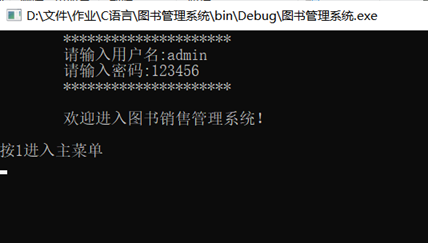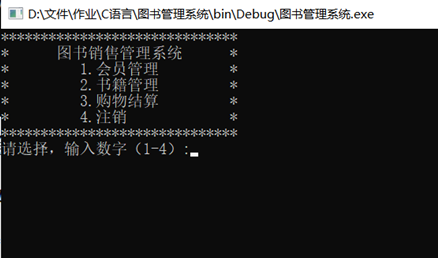2.会员管理功能
从主菜单选择会员管理后，出现会员管理的子菜单。
进入“会员管理”二级菜单，通过用户输入的操作数字1-3，进行相应的功能操作；如输入数字4，则返回“图书销售管理系统”主界面。
界面如下。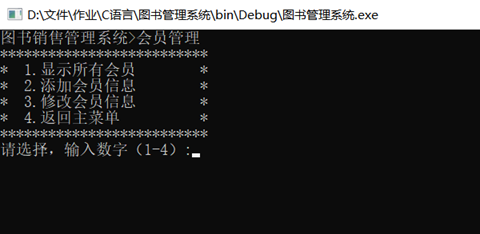（1）显示会员功能
在“会员管理”界面中，如果用户输入的是数字1，则显示如下的会员信息：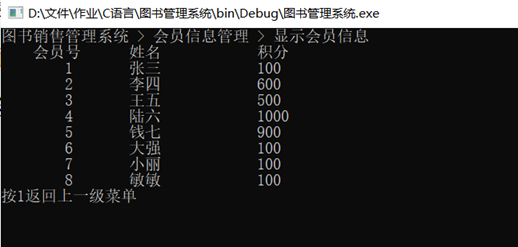（2）添加会员信息功能
返回上一级菜单后，在“会员管理”界面中，如果用户输入的是数字2，则根据用户输入的信息，进行会员添加操作（添加到现有记录的最后）。成功添加会员信息后，出现提示信息“添加成功！”。
界面如下。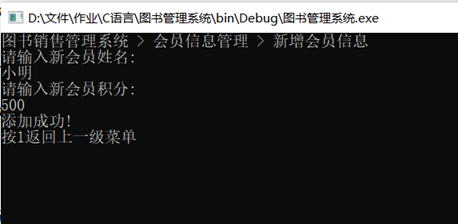按1↙返回到“会员管理”界面，此时用户可输入数字1，再次显示会员信息，查看新增加的会员信息。
界面如下。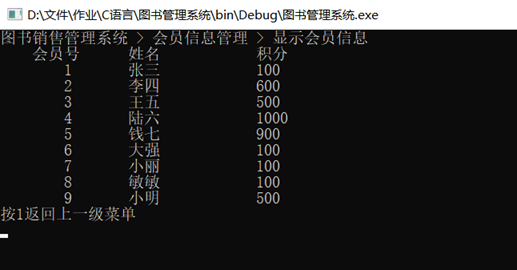可以看到小明已经成功被添加到会员列表中。

（3）修改会员信息功能
在“会员管理”界面中，如果用户输入的是数字3，则根据用户输入的会员号，进行合法性检验。
如果输入信息合法则进行会员修改操作。如果输入的会员号不存在，则显示提示信息“没找到该会员，请重新输入会员号。”然后让用户重新输入，直到输入合法为止。
修改会员信息成功后，会出现提示信息“修改成功！”
界面如下。（此处只展示合法输入的情况）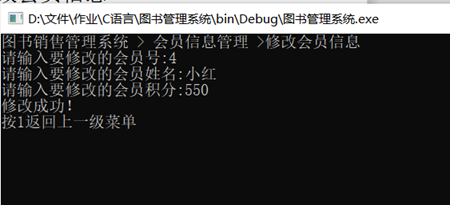按1↙返回到“会员管理”界面，此时用户可输入数字1，再次显示会员信息，以查看修改的会员信息。
界面如下。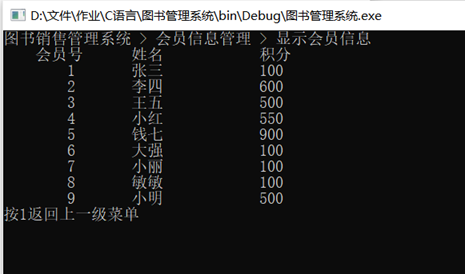可以看到会员号为4的会员信息已被成功修改。

3. 书籍管理功能
在“图书销售管理系统”主界面中，如果此时用户输入的是数字2，则进入“书籍管理”二级菜单，用户通过输入操作数字1-3，可以进行相应的功能操作；如输入数字4，则返回“图书销售管理系统”主界面。
界面如下。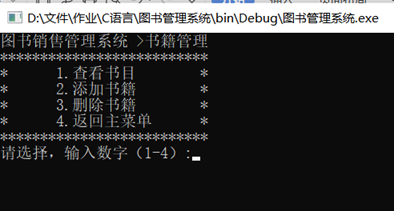（1）查看书目功能
在“书籍管理”界面中，如果用户输入的是数字1，则显示所有的图书信息。
界面如下。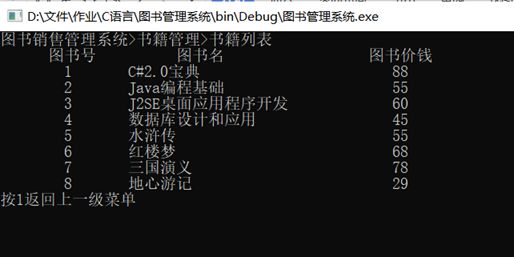（2）添加书籍功能
在“书籍管理”界面中，如果用户输入数字2，则可进行添加书籍操作（添加到现有记录的最后）。成功添加会员信息后，出现提示信息“添加成功！”。
界面如下。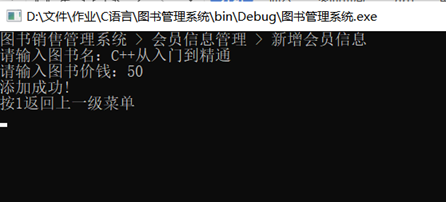按1返回“书籍管理”的二级菜单，此时用户可输入数字1，再次显示图书信息，查看新增加的书籍信息。
界面如下。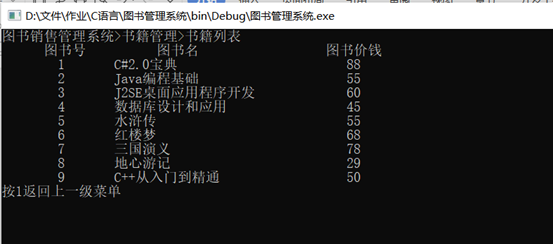可以看到书籍信息已经添加到列表末尾。

（3）删除书籍功能
在“书籍管理”界面中，如果用户输入的是数字3，则进行删除书籍操作。通过用户输入的图书名，进行合法性检验，查找该图书信息。
如果该图书存在，则输出提示信息及该图书对应的图书号“找到该图书，位置为： ”然后进行图书删除操作，成功删除后显示提示信息“删除成功！”。如果输入的图书名不存在，则显示提示信息“没找到该图书，请重新输入图书名。”然后让用户重新输入，直到输入合法为止。
界面如下。（此处只展示合法输入的情况）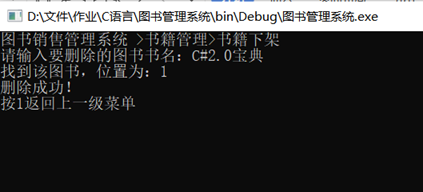返回到“书籍管理”界面，此时用户可输入数字1，再次显示图书信息，可查看删除书籍的功能效果。
界面如下。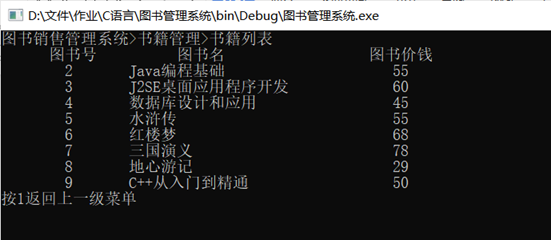可以看到C#2.0宝典已被成功删除。

4. 购物结算功能
在“图书销售管理系统”主界面中，如果此时用户输入的是数字3，则进入“购物结算”功能。
首先显示书籍的所有信息，便于用户查看购书。接着根据用户输入的会员号、书籍编号和购买量信息，并进行合法性检验。
检查用户输入会员号是否存在。如用户输入的会员号不存在，则显示提示信息“没有找到该会员，请重新输入会员号。”，并让用户重新输入，直到合法为止。
同理，检查用户输入书籍号是否存在。如用户输入的书籍号不存在，则显示提示信息“没有找到该会员，请重新输入图书号。”，并让用户重新输入，直到合法为止。
然后，检查该用户积分是否可以支付此次购书操作。如余额充足，则显示信息“您购买的图书为  ，数量为  ，总额为  。”并询问用户是否确认购买，若确认则显示“购买成功！”，否则显示“取消购买。”。
如余额不足，则提示信息“您的余额不足，购买失败。”
操作完成后，继续询问用户是否接着购书，只要用户输入的不是字符‘n’或‘N’，此购书操作可以反复进行；当输入字符‘n’或‘N’，则返回“图书销售管理系统”主界面。
界面如下。（此处只展示合法输入且购买成功的情况。）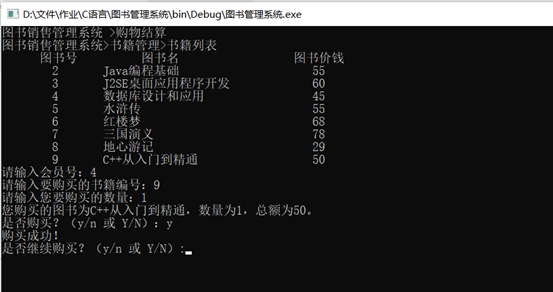此时系统返回到“图书销售管理系统”主界面，输入数字1进入“会员管理”界面，可查看刚才购书的会员信息是否发生相应的改变。
界面如下。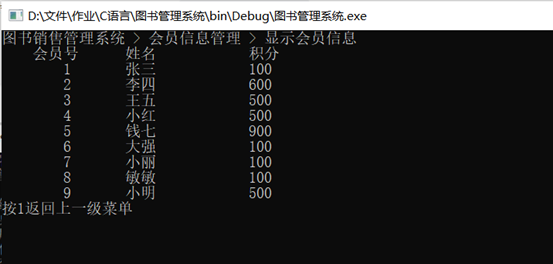可以看到会员号为4的小红，积分从550变为500。

5.注销
在主页面按4↙，释放内存，注销成功，结束程序。
界面如下。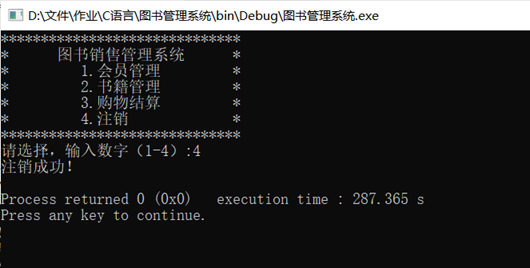源代码
#include <stdio.h>
#include <stdlib.h>
#include <string.h>
#define N 30
/*定义会员结构体*/
struct member
{
int num;
char name[N];
int points;
struct member *next;
};
/*定义书籍结构体*/
struct book
{
int num;
char name[N];
int price;
struct book *next;
};
/*登录及主菜单相关函数*/
/*会员管理相关函数*/
void MemberManagement (struct member *head,int *sum);   /*会员管理*/
struct member* ExistingMember(struct member *head,int *sum);  /*录入已有会员信息*/
void PrintMember (struct member *head);  /*打印会员信息*/
struct member* NewMember(struct member *head,int *sum);  /*新建会员信息*/
struct member* ChangeMember(struct member *head);  /*修改会员信息*/
/*书籍管理相关函数*/
struct book* ExistingBook(struct book *head,int *sum);  /*录入已有书籍信息*/
struct book* BookManagement (struct book *head2,int *sum);  /*书籍管理*/
void PrintBook (struct book *head);  /*打印书籍信息*/
struct book* NewBook(struct book *head,int *sum);  /*新建书籍信息*/
struct book* DeleteBook(struct book *head);  /*删除书籍*/
/*购物结算相关函数*/
/*释放内存*/
int main()
{
int choice;    /*菜单跳转的选项*/
int sum1=0;/*会员总数*/
int sum2=0;/*书籍总数*/
int i;
for (i=0; i<8; i++)
{
}
for (i=0; i<8; i++)
{
}
system("cls");
for(;;)
{
scanf("%d",&choice);
switch (choice)
{
case 1:
break;
case 2:
break;
case 3:
break;
case 4:
printf("注销成功！\n");
break;
}
if (choice==4) break;
}
return 0;
}
/*登录页面*/
{
int i=0,flag=0;
printf("\t*********************\n");
do
{
printf("\t请输入用户名:");
gets(user);
printf("\t请输入密码:");
if (flag==1)
{
printf("\t*********************\n");
printf("\n\t欢迎进入图书销售管理系统！\n");
int choice;
printf("\n按1进入主菜单\n");
scanf("%d",&choice);
break;
}
else
{
printf("您输入的用户名或密码错误。\n");
i++;
}
}
while(i<3);
if (flag==0)
{
system("cls");
printf("您已经输入了三次错误密码。\n");
printf("您已经退出系统。\n");
exit(0);
}
printf("*****************************\n");
}
/*验证用户输入的用户名及密码是否正确*/
{
char pass[N]="123456";    /*正确的用户名和密码*/
else return 0;
}
/*打印菜单*/
{
system("cls");
printf("******************************\n");
printf("*      图书销售管理系统      *\n");
printf("*         1.会员管理         *\n");
printf("*         2.书籍管理         *\n");
printf("*         3.购物结算         *\n");
printf("*         4.注销             *\n");
printf("******************************\n");
printf("请选择，输入数字（1-4）:");
}
/*会员管理相关函数*/
/*录入已有会员信息*/
struct member* ExistingMember(struct member *head,int *sum)
{
p=(struct member*)malloc(sizeof(struct member));
if (p==NULL)
{
printf("内存不足。\n");
exit(0);
}
{
}
else
{
while (pr->next!=NULL)
{
pr=pr->next;
}
pr->next=p;
}
(*sum)++;
p->num=*sum;
switch ((*sum))
{
case 1:
strcpy(p->name,"张三");
p->points=100;
break;
case 2:
strcpy(p->name,"李四");
p->points=600;
break;
case 3:
strcpy(p->name,"王五");
p->points=500;
break;
case 4:
strcpy(p->name,"陆六");
p->points=1000;
break;
case 5:
strcpy(p->name,"钱七");
p->points=900;
break;
case 6:
strcpy(p->name,"大强");
p->points=100;
break;
case 7:
strcpy(p->name,"小丽");
p->points=100;
break;
case 8:
strcpy(p->name,"敏敏");
p->points=100;
break;
default:
printf("录入会员信息错误。\n");
}
p->next=NULL;
}
/*会员管理*/
void MemberManagement (struct member *head1,int *sum)
{
int choice;
system("cls");
while(1)
{
scanf("%d",&choice);
switch (choice)
{
case 1:
break;
case 2:
break;
case 3:
break;
default:
break;
}
if (choice==4) break;
}
}
/*打印会员管理子菜单*/
{
system("cls");
printf("图书销售管理系统>会员管理\n");
printf("**************************\n");
printf("*  1.显示所有会员        *\n");
printf("*  2.添加会员信息        *\n");
printf("*  3.修改会员信息        *\n");
printf("*  4.返回主菜单          *\n");
printf("**************************\n");
printf("请选择，输入数字（1-4）:");
}
/*新建会员信息*/
struct member* NewMember(struct member *head,int *sum)
{
char name[N];
int points;
system("cls");
printf("图书销售管理系统 > 会员信息管理 > 新增会员信息\n");
p=(struct member*)malloc(sizeof(struct member));
if (p==NULL)
{
printf("内存不足。\n");
exit(0);
}
{
}
else
{
while (pr->next!=NULL)
{
pr=pr->next;
}
pr->next=p;
}
(*sum)++;
p->num=*sum;
printf("请输入新会员姓名:\n");
scanf("%s",name);
printf("请输入新会员积分:\n");
scanf("%d",&points);
strcpy(p->name,name);
p->points=points;
p->next=NULL;
printf("添加成功!\n");
int choice;
printf("按1返回上一级菜单\n");
scanf("%d",&choice);
}
/*打印现有会员信息*/
{
int choice;
system("cls");
printf("图书销售管理系统 > 会员信息管理 > 显示会员信息\n");
printf("    会员号  \t姓名\t\t积分\n");
do
{
printf("\t%d\t%s\t\t%d\n",p->num,p->name,p->points);
p=p->next;
}
while(p!=NULL);
printf("按1返回上一级菜单\n");
scanf("%d",&choice);
}
/*修改会员信息*/
{
system("cls");
printf("图书销售管理系统 > 会员信息管理 >修改会员信息\n");
int number,points;  /*用户输入需要修改的会员信息*/
char name[N];
int flag=0;
do
{
printf("请输入要修改的会员号:");
scanf("%d",&number);
while(p!=NULL)
{
if (p->num==number)
{
flag=1;
break;
}
p=p->next;
}
if (flag==0)
{
printf("没找到该会员，请重新输入会员号。\n");
}
else if (flag==1)
{
printf("请输入要修改的会员姓名:");
scanf("%s",name);
printf("请输入要修改的会员积分:");
scanf("%d",&points);
}
}while(flag!=1);
strcpy(p->name,name);
p->points=points;
printf("修改成功！\n");
int choice;
printf("按1返回上一级菜单\n");
scanf("%d",&choice);
}
/*书籍管理相关函数*/
/*录入已有书籍信息*/
struct book* ExistingBook(struct book *head,int *sum)
{
p=(struct book*)malloc(sizeof(struct book));
if (p==NULL)
{
printf("内存不足。\n");
exit(0);
}
{
}
else
{
while (pr->next!=NULL)
{
pr=pr->next;
}
pr->next=p;
}
(*sum)++;
p->num=*sum;
switch ((*sum))
{
case 1:
strcpy(p->name,"C#2.0宝典");
p->price=88;
break;
case 2:
strcpy(p->name,"Java编程基础");
p->price=55;
break;
case 3:
strcpy(p->name,"J2SE桌面应用程序开发");
p->price=60;
break;
case 4:
strcpy(p->name,"数据库设计和应用");
p->price=45;
break;
case 5:
strcpy(p->name,"水浒传");
p->price=55;
break;
case 6:
strcpy(p->name,"红楼梦");
p->price=68;
break;
case 7:
strcpy(p->name,"三国演义");
p->price=78;
break;
case 8:
strcpy(p->name,"地心游记");
p->price=29;
break;
default:
printf("录入书籍信息错误。\n");
}
p->next=NULL;
}
/*书籍管理*/
struct book* BookManagement (struct book *head2,int *sum)
{
int choice;
system("cls");
while(1)
{
scanf("%d",&choice);
switch (choice)
{
case 1:
system("cls");
int choice;
printf("按1返回上一级菜单\n");
scanf("%d",&choice);
break;
case 2:
break;
case 3:
break;
default:
break;
}
if (choice==4) break;
}
}
/*打印书籍管理子菜单*/
{
system("cls");
printf("图书销售管理系统 >书籍管理\n");
printf("**************************\n");
printf("*      1.查看书目        *\n");
printf("*      2.添加书籍        *\n");
printf("*      3.删除书籍        *\n");
printf("*      4.返回主菜单      *\n");
printf("**************************\n");
printf("请选择，输入数字（1-4）:");
}
/*新建书籍信息*/
struct book* NewBook(struct book *head,int *sum)
{
char name[N];
int price;
system("cls");
printf("图书销售管理系统 > 会员信息管理 > 新增会员信息\n");
p=(struct book*)malloc(sizeof(struct book));
if (p==NULL)
{
printf("内存不足。\n");
exit(0);
}
{
}
else
{
while (pr->next!=NULL)
{
pr=pr->next;
}
pr->next=p;
}
(*sum)++;
p->num=*sum;
printf("请输入图书名：");
scanf("%s",name);
printf("请输入图书价钱：");
scanf("%d",&price);
strcpy(p->name,name);
p->price=price;
p->next=NULL;
printf("添加成功!\n");
int choice;
printf("按1返回上一级菜单\n");
scanf("%d",&choice);
}
/*打印现有书籍信息*/
{
printf("图书销售管理系统>书籍管理>书籍列表\n");
printf("      图书号          图书名                  图书价钱\n");
do
{
printf("\t%d\t%-25s%10d\n",p->num,p->name,p->price);
p=p->next;
}
while(p!=NULL);
}
/*删除书籍*/
{
system("cls");
printf("图书销售管理系统 >书籍管理>书籍下架\n");
char bookname[N];    /*用户输入的图书名*/
int flag=0;
do{
printf("请输入要删除的图书书名：");
scanf("%s",bookname);
while (p!=NULL)
{
if (strcmp(p->name,bookname)==0)
{
flag=1;
printf("找到该图书，位置为：%d\n",p->num);
break;
}
p=p->next;
}
if (flag==0)
{
printf("没找到该图书，请重新输入图书名。\n");
}
}while(flag!=1);
{
free(p);
}
else
{
while (pr->next!=p)
{
pr=pr->next;
}
pr->next=p->next;
free(p);
}
printf("删除成功！\n");
int choice;
printf("按1返回上一级菜单\n");
scanf("%d",&choice);
}
/*购物结账相关的函数*/
/*购物结账*/
{
system("cls");
printf("图书销售管理系统 >购物结算\n");
int MemberNum;   /*用户会员号*/
int BookNum;   /*用户购买书本的信息*/
int amount;
int total;
while(1)
{
int flag1=0,flag2=0;
do
{
printf("请输入会员号：");
scanf("%d",&MemberNum);
while(pm!=NULL)
{
if (pm->num==MemberNum)
{
flag1=1;
break;
}
pm=pm->next;
}
if (flag1==0)
{
printf("没有找到该会员，请重新输入会员号。\n");
}
}
while(flag1!=1);
do
{
printf("请输入要购买的书籍编号：");
scanf("%d",&BookNum);
while(pb!=NULL)
{
if (pb->num==BookNum)
{
flag2=1;
break;
}
pb=pb->next;
}
if (flag2==0)
{
printf("没有找到该图书，请重新输入图书号。\n");
}
}
while(flag2!=1);
printf("请输入您要购买的数量：");
scanf("%d",&amount);
total=amount*(pb->price);
if (pm->points>=total)
{
printf("您购买的图书为%s，数量为%d，总额为%d。\n",pb->name,amount,total);
printf("是否购买？（y/n 或 Y/N）：");
char ch;
scanf(" %c",&ch);
if (ch=='n'||ch=='N')
{
printf("取消购买。\n");
}
else if (ch=='y'||ch=='Y')
{
printf("购买成功！\n");
pm->points=pm->points-total;
}
else
{
printf("没有输入y/n 或 Y/N，购买失败。");
}
}
else
{
printf("您的余额不足，购买失败。\n");
}
printf("是否继续购买？（y/n 或 Y/N）:");
char ch;
scanf(" %c",&ch);
if (ch=='n'||ch=='N')
{
break;
}
}
}
/*释放内存*/
{
while (p!=NULL)
{
pr=p;
p=p->next;
free(pr);
}
}
{
while (p!=NULL)
{
pr=p;
p=p->next;
free(pr);
}
}



有1、、想说
大一上学期结束了，可太快了……
做这个大作业的时候是期末了，猛赶ddl，代码是在一个上午（8点到11点多）加一个晚上（7点多到10点+11点多到1点）码出来的（当天中午考军事理论，当天下午出校门给室友庆生啦），然后第二天上午debug和测试，顺便把说明文档整理出来……
对于我来说，那一天真的是爆肝……
（这就是拖拉的代价）
立个flag
下学期希望能有计划地完成任务。
接下来的寒假又要爆肝了T^T
时间：2021/1/24 16:51:55


展开全文• 目录 图书销售管理系统设计 2 一设计要求 2 二总体设计思路 2 1. 主要功能系统主要包括八个功能模块 2 2. 总体思路框图 3 三主要参数和函数 4 1. 全局变量 4 2. 主要函数 4 四程序调试及整体实现 18 1. 主菜单运行...
• ## C语言课设销售管理系统设计

万次阅读 多人点赞 2016-05-26 09:21:01
该设计要求学生以某公司销售管理业务为背景，设计、开发一套“销售管理系统”软件。 通过该题目的设计过程，可以培养学生结构化程序设计的思想，加深对高级语言基本语言要素和控制结构的理解，针对c语言中的重点和...

一．          C程序设计课程设计题目简介
该设计要求学生以某公司销售管理业务为背景，设计、开发一套“销售管理系统”软件。
通过该题目的设计过程，可以培养学生结构化程序设计的思想，加深对高级语言基本语言要素和控制结构的理解，针对c语言中的重点和难点内容进行训练，独立完成有一定工作量的程序设计任务，同时强调好的程序设计风格。得到软件工程的综合训练，提高解决实际问题的能力。
二．           C程序设计课程设计的任务
1、查阅文献资料，一般在5篇以上；
2、以某公司销售管理业务为背景，通过调研、分析现有的管理模式和已有的管理软件，建立系统模型；
3、完成软件结构设计和算法设计；
4、完成系统的软件开发和测试工作；
5、撰写设计说明书；
6、做好答辩工作。
三．          C程序设计课程设计的主要内容、功能及技术指标
1、问题分析及解决方案框架确定：充分地分析和理解问题本身，弄清要求做什么。在确定解决方案框架过程中，综合考虑系统功能，考虑怎样使系统结构清晰、合理、简单和易于调试。最后确定每个过程和函数的简单功能，以及过程（或函数）之间的调用关系，并画出函数之间的调用关系图。
2、详细设计和编码：定义相应的存储结构，确定各个函数的算法，并画出流程图，在此基础上进行代码设计，每个明确的功能模块程序一般不超过60行，否则要进一步划分。
3、上机前程序静态检查：上机前程序静态检查可有效提高调试效率，减少上机调试程序时的无谓错误。静态检查主要有两种途径：用一组测试数据手工执行程序；通过阅读或给别人讲解自己的程序而深入全面地理解程序逻辑。把程序中的明显错误事先排除。
4、程序调试与测试：采用自底向上，分模块进行，即先调试低层函数。能够熟练掌握调试工具的各种功能，设计测试数据确定疑点，通过修改程序来证实它或绕过它。调试正确后，认真整理源程序及其注释，形成格式和风格良好的源程序清单和结果。
5、完成课程设计报告
① 需求和规格说明：设计题目和问题描述：题目要解决的问题是什么；
② 设计思路：主要算法思想，程序功能图，函数之间的调用关系图；
设计表示：每个函数或过程的功能，列出每个过程或函数所调用的过程或函数，并画出各函数的流程图；
详细设计：主要算法的伪代码；
③ 调试报告：调试过程中遇到的主要问题，是如何解决的；对设计和编码的回顾讨论和分析；改进设想；经验和体会等；
④ 程序实现注释；
⑤ 附录：源程序清单和结果。如果题目规定了测试数据，则结果要求包含这些测试数据和运行输出，当然还可以含其他测试数据和运行输出。
6、某公司有四个销售员（编号：1-4），负责销售五种产品（编号：1-5）。每个销售员都将当天出售的每种产品各写一张便条交上来。每张便条包含内容： 销售员的代号 、产品的代号、这种产品的当天的销售额 。每位销售员每天可能上缴0-5张便条。假设，收集到了上个月的所有便条，编写一个处理系统，读取上个月的销售情况，进行如下处理：
系统以菜单方式工作，录入信息用文件保存；计算上个月每个人每种产品的销售额； 按销售额对销售员进行排序，输出排序结果（销售员代号）；统计每种产品的总销售额，对这些产品按从高到底的顺序，输出排序结果（需输出产品的代号和销售额）；输出统计报表。下面是源代码：
#include "stdio.h" /*输入输出函数*/
#include "stdlib.h"/*通用应用程序函数*/
typedef struct/*定义结构体变量*/
{
int gonghao; /*销售员代号*/
int a1; /*产品一代号*/
int a2; /*产品二代号*/
int a3; /*产品三代号*/
int a4; /*产品四代号*/
int a5; /*产品五代号*/
}DATA;
int enter(); /*声明函数*/
void salor(int n); /*声明函数*/
int sum(DATA t,int n, int e); /*声明函数*/
void list1(int n);/*声明函数*/
void list2();/*声明函数*/
main()/*主函数*/
{      int length;
system("color 3b");

for( ; ; )/*无限循环*/
{
case 1:length=enter();break;
case 2:salor(length);break;
case 3:list1(length);break;
case 4:list2();break;
case 0:exit(0);
}
}
}
{
int n;
printf("                                    菜单                          \n\n");
printf("    设计人：吴继康、付鹏阳       班级：计算机一班\n");
printf("      1 输入便条上的内容\n");
printf("      2 统计上个月每种产品每人销售的数量\n");
printf("      3 按销售额将销售员排序\n");
printf("      4 销售报表\n");
printf("      0 退出\n");
printf("---------------------------------end---------------------------\n\n");
printf(" 请输入(0-4) ");
scanf("%d",&n);
return n;
}

int enter() /*清单输入*/
{
int i,num;
FILE *fp;
DATA t;
printf("请输入要写的便条条数：\n");
scanf("%d",&num);
printf("工号    电脑      电视      冰箱      风扇      手机");
for(i=0;i<num;i++)
{
printf("\n\n------------------------------------\n");
scanf("%d\t  %d\t  %d\t  %d\t  %d\t  %d",&t[i].gonghao,&t[i].a1,&t[i].a2,&t[i].a3,&t[i].a4,&t[i].a5);
}
if((fp=fopen("wujikang.dat","ab+"))==NULL)
{
printf("不能打开文件\n");
exit(1);
}
for(i=0;i<num;i++)
{
fprintf(fp,"%d\t%d\t%d\t%d\t%d\t%d\t",t[i].gonghao,t[i].a1,t[i].a2,t[i].a3,t[i].a4,t[i].a5);
fprintf(fp,"\r\n");
}

fclose(fp);
printf("\n-----------------------------------\n\n\n");
return i;
}
void salor(int n) /*每位销售员销售额*/
{
int i=0,s;
int b1=0,b2=0,b3=0,b4=0,b5=0;
int k=0;
DATA t;
FILE *fp;
if((fp=fopen("wujikang.dat","rb+"))==NULL)
{
printf("不能打开文件\n");
exit(1);
}
rewind(fp);
while(fscanf(fp,"%d\t%d\t%d\t%d\t%d\t%d",&(t[k].gonghao),&(t[k].a1),&(t[k].a2), &(t[k].a3),&(t[k].a4),&(t[k].a5))>0)
k++;
fclose(fp);
printf("请输入要查询售货员的代号(1-4):");
scanf("%d",&s);
for(i=0;i<k;i++)
if(t[i].gonghao==s)
{b1+=t[i].a1;
b2+=t[i].a2;
b3+=t[i].a3;
b4+=t[i].a4;
b5+=t[i].a5;};
printf("工号 电脑 电视 冰箱 风扇 手机\n");
printf("%d\t  %d\t   %d\t   %d\t       %d\t   %d\t",s,b1,b2,b3,b4,b5);

}
void list1 (int n) /*显示清单*/
{
int i,j,s;
int m,c;
int k=0;
DATA t;
FILE *fp;
if((fp=fopen("wujikang.dat","rb+"))==NULL)
{
printf("不能打开文件\n");
exit(1);
}
rewind(fp);
while(fscanf(fp,"%d\t%d\t%d\t%d\t%d\t%d",&(t[k].gonghao),&(t[k].a1),&(t[k].a2), &(t[k].a3),&(t[k].a4),&(t[k].a5))>0) /*从fp指向的文件上读入便条信息*/
k++;
fclose(fp);
for(i=0;i<4;i++) /*将所输入的条数存入结构体数组中*/
m[i]=i+1;
for(i=0;i<4;i++)
c[i]=sum(t,k,m[i]);
for(i=0;i<4;i++)
for(j=i+1;j<4;j++)
if(c[i]<c[j])
{
s=m[i];
m[i]=m[j];
m[j]=s;
s=c[i];
c[i]=c[j];
c[j]=s;
}
printf("按销售额从高到低将销售员代号排序\n");
for(i=0;i<4;i++)
printf("%d\n",m[i]);
}
void list2() /*每种产品的销售额*/
{
int i=0,j,sum0=0,sum1=0,sum2=0,sum3=0,sum4=0,sum5=0;
int c,b1={0},b2={0},b3={0},b4={0},b5={0};
int k=0;
DATA t;
FILE *fp;
if((fp=fopen("wujikang.dat","rb+"))==NULL)
{
printf("不能打开文件\n");
exit(1);
}

rewind(fp);//qingchucuowu,zhiyutoubu
while(fscanf(fp,"%d\t%d\t%d\t%d\t%d\t%d",&(t[k].gonghao),&(t[k].a1),&(t[k].a2), &(t[k].a3),&(t[k].a4),&(t[k].a5))>0)
k++;
for(i=0;i<4;i++)
c[i]=sum(t,k,i+1);/*求每个人的总销售额*/
for(i=0;i<4;i++)
sum0+=c[i]; /*所有产品的销售额*/
for(i=0;i<k;i++) /*求每个人每种产品的总销售额*/
for(j=0;j<4;j++)
{
if(j+1==t[i].gonghao)
{b1[j]+=t[i].a1;
b2[j]+=t[i].a2;
b3[j]+=t[i].a3;
b4[j]+=t[i].a4;
b5[j]+=t[i].a5;
}
}
for(i=0;i<4;i++)/*每种产品的总销售额*/
{sum1+=b1[i];
sum2+=b2[i];
sum3+=b3[i];
sum4+=b4[i];
sum5+=b5[i];
}
printf("-------------------------销售报表----------------------\n\n\n");
printf(" products num\n");
printf(" 销售员\t1\t2\t3\t4\t5\t总和\n\n");
for(i=0;i<4;i++)
printf(" %d\t%d\t%d\t%d\t%d\t%d\t%d\t\n",i+1,b1[i],b2[i],b3[i],b4[i],b5[i],c[i]);
printf("psum  %d\t%d\t%d\t%d\t%d\t%d\t",sum1,sum2,sum3,sum4,sum5,sum0);
}
int sum(DATA t,int n, int e)
{

int i=0,sum=0;
for(i=0;i<=n;i++)
if(e==t[i].gonghao)
{sum+=t[i].a1;
sum+=t[i].a2;
sum+=t[i].a3;
sum+=t[i].a4;
sum+=t[i].a5;
}
return sum;
}

展开全文C C++
• C语言写的销售管理系统，管理员功能: (1)自身密码修改 (2)用户信息管理:添加,修改,查询,删除 (3)商品信息管理:添加,修改,查询,删除 (4)销售报表显示:日报表,月报表,商品销售量报表,销售员业绩报表 (5)退出登陆系统...
• 使用.txt文件进行信息存储，使用链表 void InputBS();//1.录入待售图书信息 void SaveBS();//2.保存待售图书信息 void FindBS();//3.查找待售图书信息 void ModifyBS();//4.修改待售图书信息 ...//5....//6....
• C语言设计和实现一个图书管理系统，对图书信息进行管理。图书信息包括：图书编号(不允许重复)、图书名称、作者、出版单位、价格、数量等。 具体要求如下： (1)系统以菜单方式工作； (2)图书信息录入功能(图书信息...
• c语言的数据结构和链表实现的销售管理系统。用两个c文件实现
• C语言课程设计-产品销售管理系统C语言课程设计-产品销售管理系统C语言课程设计-产品销售管理系统，资源绝对真实可用，资源绝对真实可用，资源绝对真实可用。
• （1）录入商品信息； （2）给定商品编号，修改该商品信息；... （4）录入商品的进货与销售信息； （5）给定商品编号或商品名，查看该商品及库存信息； （6）统计功能：提供一些统计各类信息的功能。 等等功能
• C语言程序设计销售管理系统，包含多个源程序，和一些常几问题分析。
• 超市结账系统 指导老师 刘 凌 霞 班 级 2016级软件工程1班 日 期 2016年12月28日 成员及成绩 学 号 姓 名 成 绩 164804014 姬新科 164804047 岳其威 二一六年十二月 设计目的 解决问题超市需要处理大量的仓库信息...
• 他枫 険 C语言课程设计 题 目 指导教师 学生姓名 学 号 专 业 教学单位 TOC \o "1-5" \h \z \o "Current Document" 1设计内容与设计要求 1 \o "Current Document" 1.1系统功能简介 1 \o "Current Document" 1.2系统...
• 这是一篇c语言程序课程设计的完善版，我觉得题目要求的销售系统设计的功能太单一，于是我自己增加了一些功能，如销售员名字可以随意更改输入，不用仅仅输入代号；产品名称是固定的；在txt文档中增加了表头（就是每列...
• . 用户下载须知 程序语言 C C++ C# 红色字体 表示本课设使用的 程序设计语言 图形功能选项 Win32 控制台程序黑框文本界面Win32 程序MFC WinForm DirectX10 红色字体 表示本课设的 程序图形类别 默认为 非图形 界面 ...
• 介绍了基于c语言的家电销售系统，本系统主要包括的信息有8类：录入项目信息、查看项目信息、插入项目信息、修改项目信息、删除项目信息、统计库存数量、按照销量排序和按照销售额排序、有关提醒。
• 销售人员管理系统 TOC \o "1-5" \h \z 一系统描述 2 \o "Current Document" 二系统设计 2 \o "Current Document" 三详细设计 2 1存储结构 2 mai n)主函数 3 登陆界面 3 add)t曾加函数(编辑) 4 ame nd(修改函数 4 ...
• 销售管理系统报告书 c语言每一记录包括销售员的编号、产品编号、产品当天的销售额、上个月每个人每种产品的销售额、每种产品的总销售额。...

# c语言销售管理系统c语言 订阅